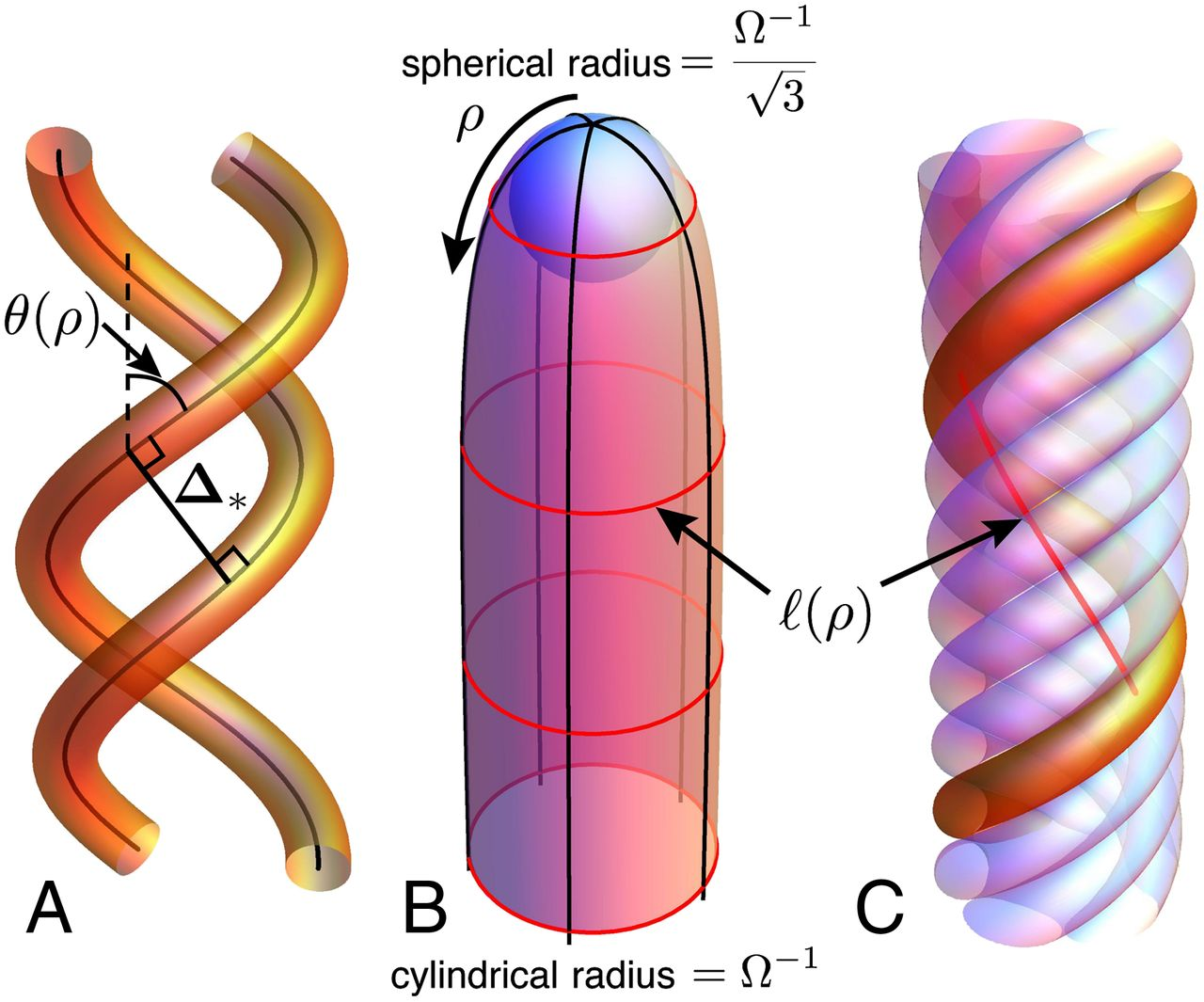# Rotation vs Revolution

``````---+-----+-----
1 | {1} | {2}
---+-----+-----
2 |  3  | 101
---+-----+-----
3 |{102}| 111
---+-----+-----
``````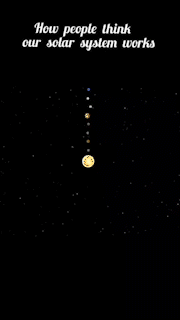In mathematics, a tensor is an algebraic object that describes a multilinear relationship between sets of algebraic objects related to a vector space. Tensors may map between different objects such as vectors, scalars, and even other tensors.

In some areas, tensor fields are so ubiquitous that they are often simply called "tensors". Tullio Levi-Civita and Gregorio Ricci-Curbastro popularised tensors in 1900 – continuing the earlier work of Bernhard Riemann and Elwin Bruno Christoffel and others – as part of the absolute differential calculus. The concept enabled an alternative formulation of the intrinsic differential geometry of a manifold in the form of the Riemann curvature tensor (Wikipedia).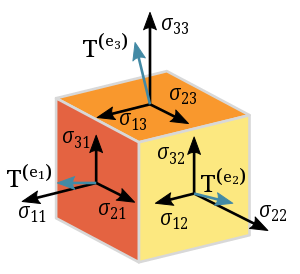A Riemann surface is a "universe" locally modelled on open sets in the complex plane, and equipped with extra structure so that complex analysis can be done. Polynomial equations in two variables define special Riemann surfaces, called plane curves, such asthe "circle" z2 + w2 = 1, ( rotating the w-plane), the "cubic" z3 + w2 = 1, the "nodal cubic" z3 + z2 = w2, and the "Fermat quartic" z4 + w4 = 1 (Pinterest).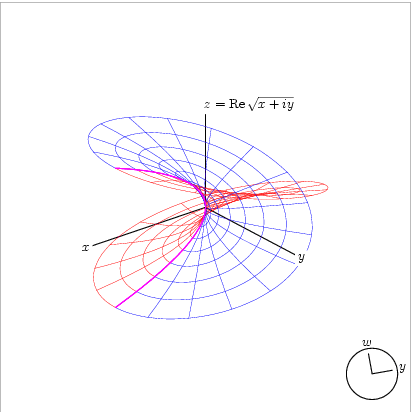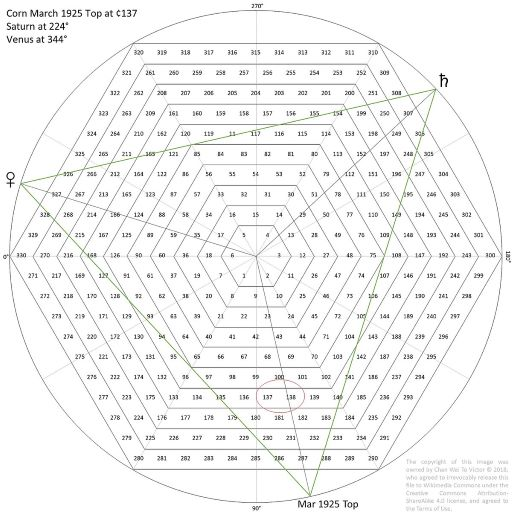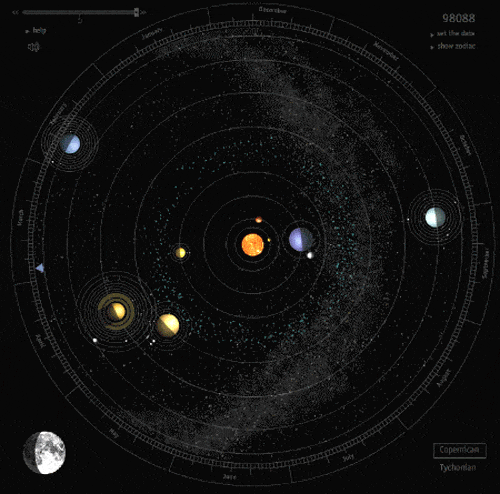## Moon's orbit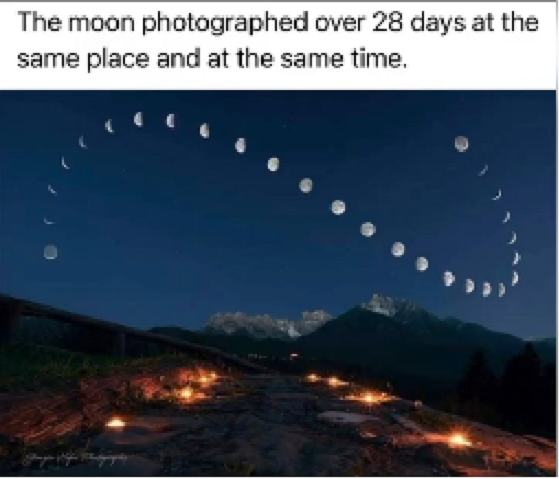``````1 year = 12 months
1000 years = 12,000 months

c = 12,000 x L / t
= 12,000 x 2152612.336257 km / 86164.0906 sec
= 299,792.4998 km / sec

Note:
Te = earth revolution = 365,25636 days
R = radius of moon rotation to earth = 384,264 km
V = moon rotation speed = 2πR/Tm = 3682,07 km/hours
Ve = excact speed = V cos (360° x Tm/Te) = V cos 26,92848°
Tm = moon revolution (sidereal) = 27,321661 days = 655,719816 hours
t = earth rotation (sinodik) = 24 hours = 24 x 3600 sec = 86164.0906 sec
L = Ve x Tm = 3682,07 km/hours x cos 26,92848° x 655,71986 = 2152612.336257 km
``````

So by the theory of E=mc² to raise up a particle on earth it should be a groups of galaxies that rationaly and irrationaly parallel to its position in our Solar system. It was predicted that we have 1012 stars in our galaxy and 2×1012 galaxies in the universe.

In 2016, using 20 years of images from the Hubble space telescope, it was estimated that there were in total two trillion (2×1012) or more galaxies in the observable universe, and as many as an estimated 1×1024 stars (more stars than all the grains of sand on all beaches of the planet Earth) (Wikipedia)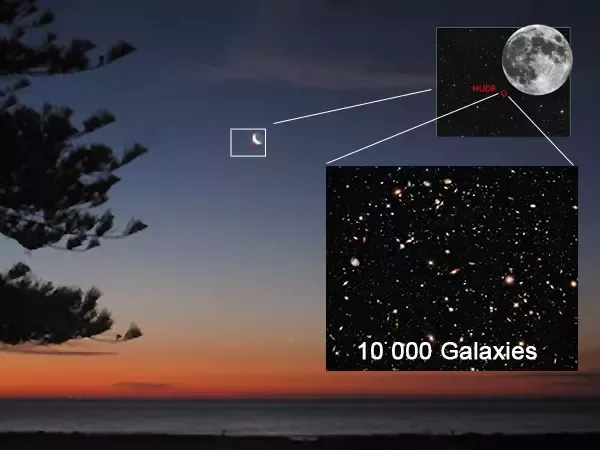Recent observation and a census from NASA Hubble Telescope together with other observatories show that observable Universe may contain 10 times more Galaxies than previous estimates! So remember, this number won't get the end story.

286 - (231x5)/(11x7) = 286 - 1155/77 = 286 - 15 = 200 + 71 = 271

``````-----+-----+-----+-----+-----+                                               ---
19¨ |  3¨ |  4¨ |  6¨ |  6¨ | 4¤                                             |
-----+-----+-----+-----+-----+                                                |
17¨ | {5¨}| {3¨}|  2¨ |  7¨ | 4¤                                             |
+-----+-----+-----+-----+                                                |
{12¨}|  6¨ |  6¨ |  2¤ (M dan F)                                              |
+-----+-----+-----+                                                     17¤
11¨ |  3¨ | {3¨}| {5¨}| 3¤                                                   |
-----+-----+-----+-----+-----+                                                |
19¨ |  4¨ |  4¨ |  5¨ |  6¨ | 4¤                                             |
+-----+-----+-----+-----+                                               ---
{18¨}|  5¨ |  5¨ |  8¨ | 3¤                                                   |
+-----+-----+-----+-----+-----+-----+-----+-----+-----+                 12¤
43¨ | {3¨}| {5¨}|  5¨ | {5¨}| {3¨}|  7¨ | {5¨}| {3¨}|  7¨ | 9¤ (C1 dan C2)   |
-----+-----+-----+-----+-----+-----+-----+-----+-----+-----+                 ---
139¨ |-----  13¨  -----|------ 15¨ ------|------ 15¨ ------|
|  1     2     3  |  4     5     6  |  7     8     9  |
Δ                 Δ                 Δ
``````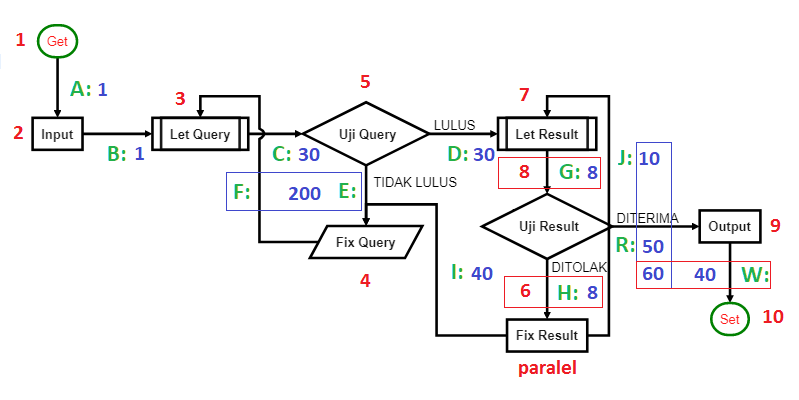``````layer | node | sub |    i     |   f
------+------+-----+----------+-----+-----+-----+                                    ---
|      |     |    1,2:1 |   1 |  30 |  40 | 71 (2,3) ‹-------------------       |
|      |  1  +----------+-----+-----+-----+                              |      |
|  1   |     |        2 |                                                |      5¨  encapsulation
|      |-----+----------+            -----------------------------       |      |
|      |     |        3 |           |                             |      |      |
1   +------+  2  +----------+----       |       LAGGING SCHEME        |      |     ---
|      |     |        4 |           |    (Exponentiation Zone)    |      |      |
|      +-----+----------+           |                             |      |      |
|  2   |     |        5 |           ------------------------------       |      7¨  abstraction
289   |      |  3  +----------+                                                |      |
|     |      |     |        6 |  ‹---------------------------- Φ               | {6®} |
------+------+-----+----------+-----+-----                                     |     ---
|      |     |     11:7 |   5 |   9 |  14 (20) --------› ¤               |      |
|      |  4  +----------+-----+-----+-----+                              |      |
|  3   |     |     12:8 |   9 |  60 |  40 | 109 (26) «------------       |     11¨  polymorphism
|      +-----+----------+-----+-----+-----+                       |      |      |
|      |     |     13:9 |   9 |  60 |  69 (27) «-- Δ19 (Rep Fork) | {2®} |      |
2   +------|  5  +----------+-----+-----+-----+                       |      |     ---
|      |     |    14:19 |   9 |  60 |  40 | 109 (28) -------------       |      |
|      |-----+----------+-----+-----+-----+                              |      |
|  4   |     | 15,18:11 |   1 |  30 |  40 | 71 (29,30,31,32) ------------      13¨  inheritance
329   |      |  6  +----------+-----+-----+-----+                                     |
|     |      |     |    19:12 |  10 |  60 | {70} (36) -------› Φ                      |
------+------+-----+----------+-----+-----+                                          ---
``````